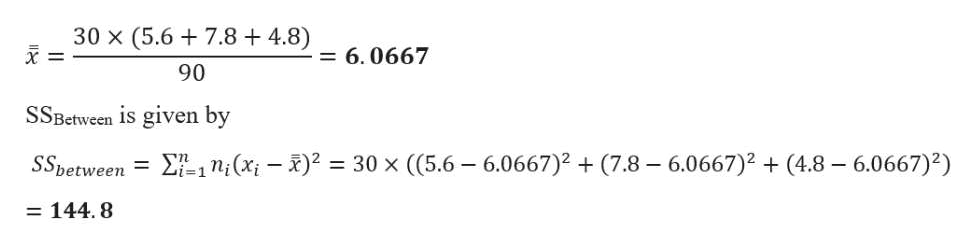# If the sample size is 30 and the mean score for football players are 5.6, mean score for basketball players are 7.8, and mean score for baseball players are 4.8, what is the SS between of this study?

Question
4 views

If the sample size is 30 and the mean score for football players are 5.6, mean score for basketball players are 7.8, and mean score for baseball players are 4.8, what is the SS between of this study?

check_circle

Step 1

Assuming The sample size is 30 for each group

Given data and calculation is shown below

Step 2

Grand mean is give...help_outlineImage Transcriptionclose30 x (5.67.8 + 4.8) = 6.0667 90 SSBetween is given by 1 n (x)2 30 x ( (5.6 6.0667)(7.8-6.0667)2(4.8-6.0667)2) SSbetween = = 144. 8 fullscreen

### Want to see the full answer?

See Solution

#### Want to see this answer and more?

Solutions are written by subject experts who are available 24/7. Questions are typically answered within 1 hour.*

See Solution
*Response times may vary by subject and question.
Tagged in

### Other